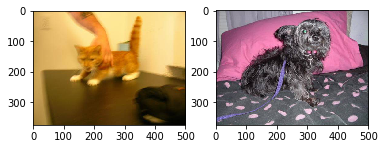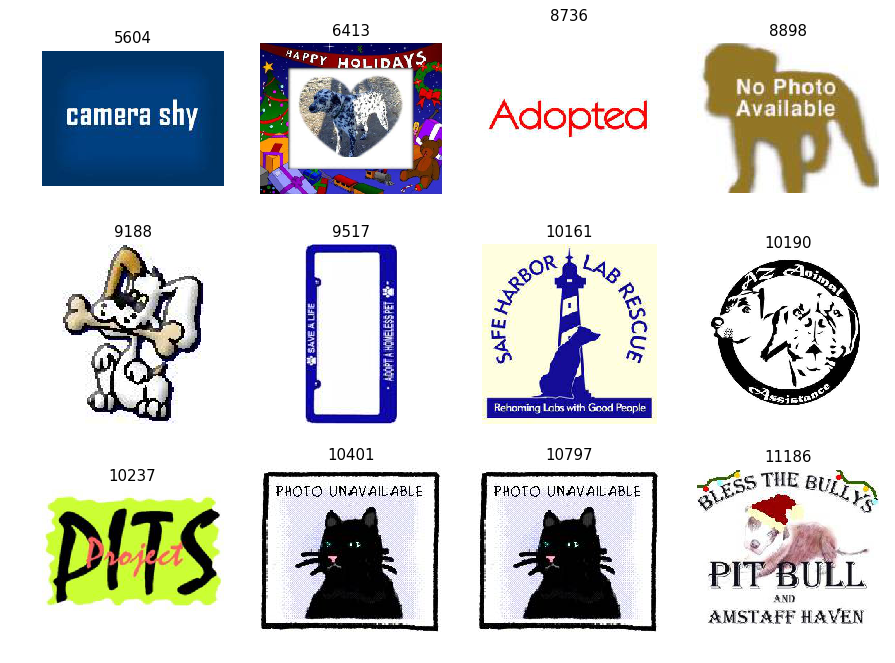# Image Recognition: Dogs vs Cats! (92%)

Classify dog and cat pictures with a 92% accuracy with a deep convolutional neural network.

## Introduction

In this article, you will build a convolutional neural network from scratch to classify images into two categories, dog or cat, with a 92% accuracy.

We're not going to use transfer learning this time (so no cheating!), and I will explain in details the process I followed to solve this classic problem.

You will learn how to:

• build and tune a convolutional network with keras for image classification
• choose the right optimizer to make sure that your network is able to learn
• use the keras ImageDataGenerator to augment your dataset and limit overfitting

Finally, you will try the ResNet50 pre-trained model, just to see.

## Running this tutorial ¶

Unlike most posts on this blog, I do not provide a recipe to run this notebook on Google Colab. I tried it, but it appears that:

• the disk transfers on the Google Colab virtual machines are too slow. This very much slows down the training of neural networks on relatively large datasets such as the dogs and cats dataset.
• the number of CPU cores on these machines is too low to handle the heavy image preprocessing that we will need to apply.

As a consequence, you'll need to run on your machine.

First, install TensorFlow for your Linux or Windows PC. With these recipes, you will also install anaconda, including the keras package.

Then, install these packages with conda:

``conda install numpy matplotlib``

Clone my github repo locally, and start the notebook:

``````git clone https://github.com/cbernet/maldives.git
cd maldives/dogs_vs_cats
jupyter notebook``````

And open the notebook ``` dogs_vs_cats_local.ipynb ``` .

I haven't tested this recipe, so if it doesn't work, tell me in the comments and I'll help right away.

## The dogs and cats dataset ¶

The dogs and cats dataset was first introduced for a Kaggle competition in 2013. To access the dataset, you will need to create a Kaggle account and to log in. No pressure, we're not here for the competition, but to learn!

The dataset is available here . You can use the kaggle utility to get the dataset, or simply download the train.zip file (about 540 MB). Don't forget to log in to Kaggle first.

The instructions to prepare the dataset are for Linux or macOS. If you work on Windows, I'm sure you can easily find a way to do that (e.g. use 7-zip do unpack the archive, and Windows Explorer to create directories and move files around).

``unzip train.zip``

List the contents of the train directory:

``ls train``

You will see a lot of images of dogs and cats.

In the next sections, we will use Keras to retrieve images from disk with the flow_from_directory method of the ImageDataGenerator class.

This method however requires the images from the different classes to be sorted in different directories. So we will put all dog images in ``` dogs ``` , and all cat images in ``` cats ``` :

``````mkdir cats
mkdir dogs
find train -name 'dog.*' -exec mv {} dogs/ \;
find train -name 'cat.*' -exec mv {} cats/ \;``````

You might be wondering why I used ``` find ``` instead of a simple ``` mv ``` to move the files. It's because with ``` mv ``` , the shell needs to pass a very large number of arguments to the command (all the file names), and there is a limitation on this number on macOS (on Linux, it's working fine). With ``` find ``` , we can work around this limitation.

## Initialization ¶

Now, enter in the cell below the location of your dataset directory, the one that contains the ``` dogs ``` and ``` cats ``` subdirectories, and execute it:

In :
```# define and move to dataset directory
datasetdir = '/data2/cbernet/maldives/dogs_vs_cats'
import os
os.chdir(datasetdir)

# import the needed packages
import matplotlib.pyplot as plt
import matplotlib.image as img
from tensorflow import keras
# shortcut to the ImageDataGenerator class
ImageDataGenerator = keras.preprocessing.image.ImageDataGenerator
```

## A first look at the dogs and cats dataset ¶

We can start investigating the dataset by plotting the first picture in each category:

In :
```plt.subplot(1,2,1)
plt.subplot(1,2,2)
```
Out:
`<matplotlib.image.AxesImage at 0x7f33ea1b7b38>`Cute. But let's be more specific and print some information about our images:

In :
```images = []
for i in range(10):
images.append(im)
print('image shape', im.shape, 'maximum color level', im.max())
```
```image shape (374, 500, 3) maximum color level 255
image shape (280, 300, 3) maximum color level 255
image shape (396, 312, 3) maximum color level 255
image shape (414, 500, 3) maximum color level 255
image shape (375, 499, 3) maximum color level 255
image shape (144, 175, 3) maximum color level 255
image shape (303, 400, 3) maximum color level 255
image shape (499, 495, 3) maximum color level 255
image shape (345, 461, 3) maximum color level 255
image shape (425, 320, 3) maximum color level 247
```

In the image shape, the first two columns correspond to the height and width of the image in pixels, respectively, and the third one to the three color channels. So each pixel contains three values (for red, green, and blue, respectively). We also print the maximum color level in each channel, and we can conclude that the RGB levels are in the range 0-255.

## Pet cleaning: improving the dataset quality ¶

If there is only one thing that you should take away from this tutorial it is this:

You Should Never Trust Your Data

The data are always dirty.

To get a close look at this dataset, I used a fast image browser to check all images in the dogs and cats directories. Actually, I simply used the Preview application on my mac to browse through the small preview icons. The brain is very fast to spot obvious issues even if you just let your eyes wander on a large number of pictures. So this (tedious) work took me no more than 20 minutes. But of course, I have certainly missed a lot of less obvious issues.

Anyway, here is what I found.

First, here are the indices for the bad images I could find in each category:

In :
```bad_dog_ids = [5604, 6413, 8736, 8898, 9188, 9517, 10161,
10190, 10237, 10401, 10797, 11186]

bad_cat_ids = [2939, 3216, 4688, 4833, 5418, 6215, 7377,
8456, 8470, 11565, 12272]
```

We can then retrieve the images with these ids from the ``` cats ``` and ``` dogs ``` directories:

In :
```def load_images(ids, categ):
'''return the images corresponding to a list of ids'''
images = []
dirname = categ+'s' # dog -> dogs
for theid in ids:
fname = '{dirname}/{categ}.{theid}.jpg'.format(
dirname=dirname,
categ=categ,
theid=theid
)
images.append(im)
return images
```
In :
```bad_dogs = load_images(bad_dog_ids, 'dog')
```
In :
```def plot_images(images, ids):
ncols, nrows = 4, 3
fig = plt.figure( figsize=(ncols*3, nrows*3), dpi=90)
for i, (img, theid) in enumerate(zip(images,ids)):
plt.subplot(nrows, ncols, i+1)
plt.imshow(img)
plt.title(str(theid))
plt.axis('off')
```
In :
```plot_images(bad_dogs, bad_dog_ids)
```Some of these images are completely meaningless, like 5604 and 8736. For 10401 and 10797, we actually see a cat in the picture! sigh... Keeping or not cartoon dogs is debatable. My feeling is that it's going to be better to remove them. Same for 6413, we could keep it, but I'm afraid that the network would focus on the drawings around the dog picture.

Now let's look at the bad cats:

In :
```plot_images(bad_cats, bad_cat_ids)
```